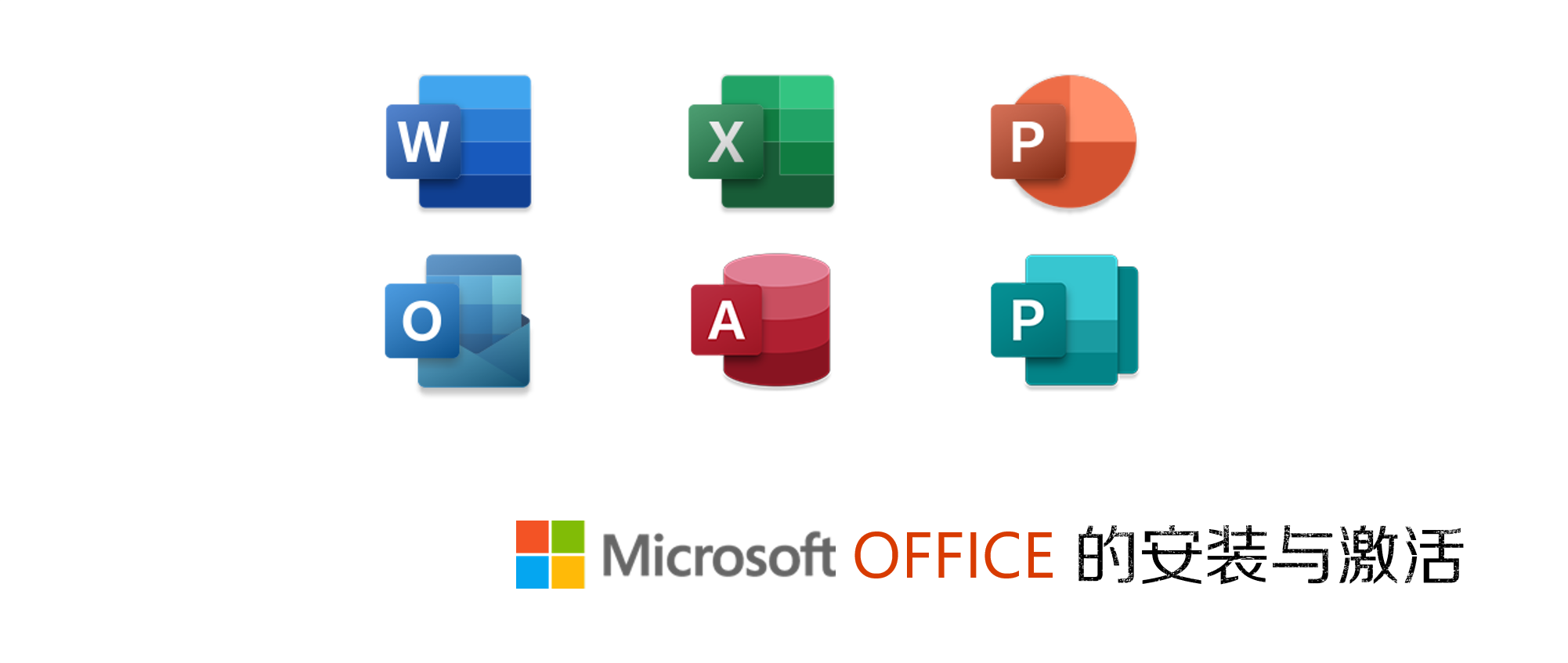# Java 接口# 2、接口的作用

• 是面向对象的一个重要机制
• 是继承多个设计
• 建立了类和类之间的“协议”：
• 将类根据其实现的功能分组用接口代表，而不必顾虑它所在的类继承层次；这样可以最大限度地利用动态绑定，隐藏实现细节
• 实现不同类之间的常量共享
• 接口允许我们在看起来不相干的对象之间定义共同行为，如下UML类图所示：# 3、接口的语法

``````[接口修饰符] interface 接口名称 [extends 父接口名]{
//方法的原型声明或静态常量
}``````

• 接口的数据成员一定要有初值，且此值将不能再更改，可以省略`final`关键字，即接口中每一个方法是隐式抽象的，接口中的方法被隐式的指定为 `public abstract`（只能是`public abstract`，其他修饰符都会报错）；
• 接口中的方法必须是抽象方法，不能有方法体，可以省略`public``abstract`关键字，即接口中的变量会被隐式的指定为`public static final`变量（并且只能是`public`，用`private`修饰会报编译错误）。

# 4、接口与类的区别

• 接口不能用于实例化对象。
• 接口没有构造方法。
• 接口中所有的方法必须是抽象方法，只能由实现接口的类来实现接口中的方法。
• 接口不能包含成员变量，除了`static``final`变量。
• 接口不是被类继承了，而是要被类实现
• 接口支持多继承

# 5、抽象类和接口的区别

• 抽象类中的方法可以有方法体，就是能实现方法的具体功能，但是接口中的方法不行。

注：JDK 1.8 以后，接口里可以有静态方法和方法体了。

• 抽象类中的成员变量可以是各种类型的，而接口中的成员变量只能是`public static final`类型的。

• 一个类只能继承一个抽象类，而一个类却可以实现多个接口。

• 接口是隐式抽象的，当声明一个接口的时候，不必使用`abstract`关键字。
• 接口中每一个方法也是隐式抽象的，声明时同样不需要`abstract`关键字。
• 接口中的方法都是公有的。

``````interface Shape2D {    //声明Shape2D接口
final double pi = 3.14;            //数据成员一定要初始化
public abstract double area();    //抽象方法
}``````

``````interface Shape2D {
double pi = 3.14;
double area();
}``````

# 6、实现接口

## (1) 单一接口的实现

``````public class 类名称 implements 接口名称 {
//在类体中实现接口的方法
//本类声明的更多变量和方法
}``````

• 必须实现接口中的所有方法；
• 来自接口的方法必须声明成`public`

``````class Circle implements Shape2D {
public Circle(double r) {
}
public double area() {
}
}
class Rectangle implements Shape2D {
int length, width;
public Rectangle(int l, int w) {
length = l;
width = w;
}
public double area() {
return (width * length);
}
}
//测试类
public class InterfaceTester {
public static void main(String args[]) {
Rectangle rect = new Rectangle(5, 6);
System.out.println("Area of rect = " + rect.area());
Circle cir = new Circle(2.0)
System.out.println("Area of cir = " + cir.area());
}
}``````

``````Area of rect = 30.0
Area of cir = 12.56``````

## (2) 多个接口的实现

Java不允许一个类有多个超类

``````[类修饰符] class 类名称 implements 接口1, 接口2, … {
//在类体中实现接口的方法
//本类声明的更多变量和方法
}``````

1. 重写接口中声明的方法时，需要注意以下规则：
• 类在实现接口的方法时，不能抛出强制性异常，只能在接口中，或者继承接口的抽象类中抛出该强制性异常。
• 类在重写方法时要保持一致的方法名，并且应该保持相同或者相兼容的返回值类型
• 如果实现接口的类是抽象类，那么就没必要实现该接口的方法
1. 在实现接口的时候，也要注意一些规则：
• 一个类只能继承一个类，但是能实现多个接口
• 一个接口能继承另一个接口，这和类之间的继承比较相似。

# 7、接口类型的引用变量

``````public class VariableTester {
public static void main(String args[]) {
Shape2D var1, var2;
var1 = new Rectangle(5, 6);
System.out.println("Area of var1 = " + var1.area());
var2 = new Circle(2.0);
System.out.println("Area of var2 = " + var2.area());
}
}``````

``````Area of rect = 30.0
Area of cir = 12.56``````

# 8、接口的扩展

• 原来的接口称为超接口（super interface）；
• 派生出的接口称为子接口（sub interface）。

``````interface 子接口的名称 extends 超口的名称1, 超接口的名称2, … {
//……
}````````````//接口声明
//声明Shape接口
interface Shape {
double pi = 3.14159;
void setColor(String str);
}
//声明Shape2D接口扩展了Shape接口
interface Shape2D extends Shape {
double area();
}
//声明Shape3D接口扩展了Shape接口
interface Shape3D extends Shape {
double superficialArea();
double volume();
}

//类声明
//Circle类实现了Shape2D接口
class Circle implements Shape2D {
String color;
public Circle(double r) {
color = "white";
}
public double area() {
}
public void setColor(String str) {
color = str;
System.out.println("color = " + color);
}
}
//Rectangle类实现了Shape2D接口
class Rectangle implements Shape2D {
int length, width;
String color;
public Rectangle(int l, int w) {
length = l;
width = w;
color = "white";
System.out.println("New Rectangle: length = " + length
+ ", width = " + width);
}
public double area() {
return (width * length);
}
public void setColor(String str) {
color = str;
System.out.println("color = " + color);
}
}
//Box类实现了Shape3D接口
class Box implements Shape3D {
int length, width, height;
String color;
public Box(int l, int w, int h) {
length = l;
width = w;
height = h;
color = "white";
System.out.println("New Rectangle: length = " + length
+ ", width = " + width + ", height = " + height);
}
public double superficialArea() {
return (2 * length * width + 2 * height * width + 2 * length * height);
}
public double volume() {
return (length * width * height);
}
public void setColor(String str) {
color = str;
System.out.println("color = " + color);
}
}
//Sphere类实现了Shape3D接口
class Sphere implements Shape3D {
String color;
public Sphere(double r) {
color = "white";
}
public double superficialArea() {
}
public double volume() {
}
public void setColor(String str) {
color = str;
System.out.println("color = " + color);
}
}

//测试类
public class ExtendsInterfaceTester {
public static void main(String[] args) {
Circle cir;
cir = new Circle(2.0);
cir.setColor("blue");
System.out.println("Area of Circle cir is " + cir.area());
System.out.println();

Rectangle rec;
rec = new Rectangle(5, 6);
rec.setColor("red");
System.out.println("Area of Rectangle rec is " + rec.area());
System.out.println();

Box box;
box = new Box(3, 4, 5);
box.setColor("green");
System.out.println("Superficial Area of Box box is " + box.superficialArea());
System.out.println("Volume of Box box is " + box.volume());
System.out.println();

Sphere sph;
sph = new Sphere(15.0);
sph.setColor("yellow");
System.out.println("Superficial Area of Sphere sph is " + sph.superficialArea());
System.out.println("Volume of Sphere sph is " + sph.volume());
System.out.println();
}
}``````

``````New Circle: radius = 2.0
color = blue
Area of Circle cir is 12.56636

New Rectangle: length = 5, width = 6
color = red
Area of Rectangle rec is 30.0

New Rectangle: length = 3, width = 4, height = 5
color = green
Superficial Area of Box box is 94.0
Volume of Box box is 60.0

color = yellow
Superficial Area of Sphere sph is 2827.431
Volume of Sphere sph is 14137.155
``````

# 9、标记接口 △

``````package java.util;
public interface EventListener {}``````

• 建立一个公共的父接口：

正如`EventListener`接口，这是由几十个其他接口扩展的Java API，你可以使用一个标记接口来建立一组接口的父接口。例如：当一个接口继承了`EventListener`接口，Java虚拟机(JVM)就知道该接口将要被用于一个事件的代理方案。

• 向一个类添加数据类型：

这种情况是标记接口最初的目的，实现标记接口的类不需要定义任何接口方法（因为标记接口根本就没有方法），但是该类通过多态性变成一个接口类型。

上一篇详细教程 | Office的安装与激活
Office作为一款常用的办公软件，在我们的学习和工作中是不可少的。然而，这款软件的安装和激活困扰了很多人。下面用这三个方案，试试看能不能解决你的问题。
2020-03-27Java 类的重用

2020-03-18
目录